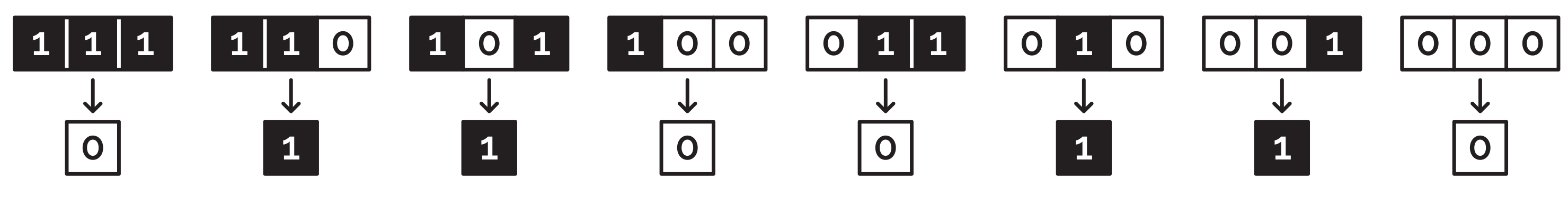# On the cover: cellular automata

Discover the meaning of the coloured squares on the cover of issue 13The game of life—invented by John Conway in 1970—is perhaps the most famous cellular automaton. Cellular automata consist of a regular grid of cells (usually squares) that are (usually, see the end of this article) either ‘on’ or ‘off’. From a given arrangement of cells, then the state of each cell in the next generation can be decided by following a set of simple rules. Surprisingly complex patterns can often arise from these simple rules.

While the game of life uses a two-dimensional grid of squares for each generation, the cellular automaton on the cover of this issue of Chalkdust is an elementary cellular automaton: it uses a one-dimensional row of squares for each generation. As each generation is a row, subsequent generations can be shown below previous ones.

## Elementary cellular automataAn example rule

In an elementary cellular automaton, the state of each cell is decided by its state and the state of its two neighbours in the previous generation. An example such rule is shown to the right: in this rule, the a cell will be on in the next generation if it and its two neighbours are on–off–on in the current generation. A cellular automaton is defined by eight of these rules, as there are eight possible states of three cells.

In 1983, Stephen Wolfram proposed a system for naming elementary cellular automata. If on cells are 1 and off cells are 0, all the possible states of three cells can be written out (starting with 1,1,1 and ending 0,0,0). The states given to each middle cell in the next generation gives a sequence of eight ones and zeros, or an eight-digit binary number. Converting this binary number into decimal gives the name of the rule. For example, rule 102 is shown below.Rule 102: so called because (0)1100110 is 102 in binary

Rule 102 is, in fact, the rule that created the pattern shown on the cover of this issue of Chalkdust. To create a pattern like this, first start with a row of squares randomly assigned to be on or off:You can then work along the row, working out whether the cells in the next generation will be on or off. To fill in the end cells, we imagine that the row is surrounded by an infinite sea of zeros.… and so on until you get the full second generation:If you continue adding rows, and colour in some of the regions you create, you will eventually get something that looks like this:It’s quite surprising that such simple rules can lead to such an intricate pattern. In some parts, you can see that the same pattern repeats over and over, but in other parts the pattern seems more chaotic.

The pattern gets a square wider each row. This is due to the state 001 being followed by 1: each new 1 from this rule will lead to another 1 that is one square further left.

But just when you think you’re getting used to the pattern of some small and some slightly larger triangles…Surprise! There’s this huge triangle that appears out of nowhere.

## Other rules

Rule 102 is of course not the only rule that defines a cellular automaton: there are 256 different rules in total.

Some of these are particularly boring. For example, in rule 204 each generation is simply a copy of the previous generation. Rule 0 is a particularly dull one too, as after the first generation every cell will be in the off state.Rule 204 is one of the most boring rules as each new cell is a copy of the cell directly above it.

Some other rules are more interesting. For example, rules 30 and 150 make interesting patterns.100 rows of rule 30 starting with a row of 100 cells in a random state100 rows of rule 150 starting with a row of 100 cells in a random state

If you want to have a go at creating your own cellular automaton picture, you can use this handy template. If you’d rather get a computer to do the colouring for you, you can download the Python code I wrote to create the pictures in this article and try some rules out.

There are also many ways that you can extend the ideas to create loads of different automata. For example, you could allow each cell to be in one of three states (‘on’, ‘off’, or ‘scorpion’) instead of the two we’ve been allowing. You could then choose a rule assigning one of the three states to each of the 27 possible configurations that three neighbouring three-state cells could be in. But there are 7,625,597,484,987 different automata you could make in this way, so don’t try to draw them all…Matthew is a postdoctoral researcher at University College London. He hasn’t had time to play Klax since the noughties, but he’s pretty sure that Coke is it!